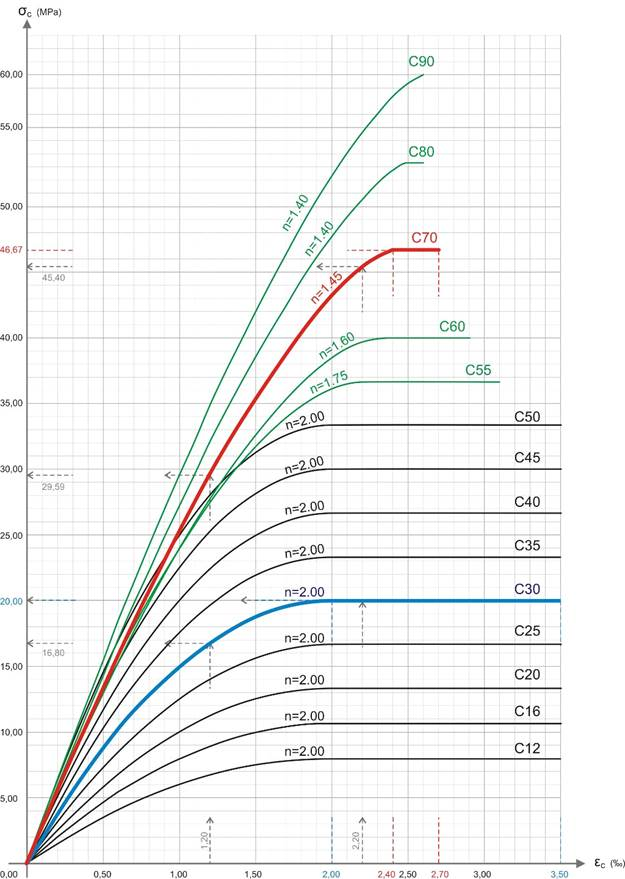# Concrete and SteelIntroduction >
The structural frame
The construction
The reinforcement I
The reinforcement II
Quantity/Cost estimation
Detailing drawingsIntroduction >
Wind and Seismic Forces >
Structural model and Analysis
Modelling slabs
Slabs
Seismic behavour of frames
Appendix A
Appendix B
Appendix C
Appendix DIntroduction >
Materials
To be continued >

## ConcreteStress-strain diagrams for each concrete strength class
for acc=1.00 and γc=1.50, where n is the exponent of each curve.

## Table 3.1: Characteristic compressive strength and concrete deformation [EC2, 3.1.2(3)]

 Strength Class C12 C16 C20 C25 C30 C35 C40 C45 C50 C55 C60 C70 C80 C90 fck (MPa) 12 16 20 25 30 35 40 45 50 55 60 70 80 90 fck,cube (MPa) 15 20 25 30 37 45 50 55 60 67 75 85 95 105 fcm (MPa) 20 24 28 33 38 43 48 53 58 63 68 78 88 98 fctm (MPa) 1,6 1,9 2,2 2,6 2,9 3,2 3,5 3,8 4,1 4,2 4,4 4,6 4,8 5,0 fctk,0.05 (MPa) 1,1 1,3 1,5 1,8 2,0 2,2 2,5 2,7 2,9 3,0 3,1 3,2 3,4 3,5 fctk,0.95 (MPa) 2,0 2,5 2,9 3,3 3,8 4,2 4,6 4,9 5,3 5,5 5,7 6,0 6,3 6,6 Ecm (GPa) 27 29 30 31 33 34 35 36 37 38 39 41 42 44 εc2 (‰) 2,0 2,2 2,3 2,4 2, 5 2,6 εcu2 (‰) 3,5 3,1 2,9 2,7 2,6 2,6 n 2,0 1,75 1,6 1,45 1,4 1,4

Concrete strength classes are determined by the Characteristic Compressive Cylinder Strength fck of concrete at 28 days , with a maximum permissible value of Cmax=90 [EC2, 3.1.2(2)A].

The characteristic compressive strengths fck and the corresponding mechanical properties required by design are presented in the following table 3.1 [EC2, 3.1.2(3)] & [EN 206-1].

The strength parameters for each concrete class are:

fck            Characteristic compressive cylinder strength of concrete at 28

fck,cube     Characteristic compressive cube strength of concrete at 28 days, according to EN 206-1

fcm           Mean value of concrete cylinder compressive ,  fcm = fck + 8 (MPa)

e.g. for C20, fcm = 20+8=28 MPa

fctm                    Mean value of axial tensile strength of concrete,

fctm = 0,30×fck2/3 ≤ C50/60 andι fctm=2,12×ln(1+fcm/10) > C50/60

e.g. for C20, fctm=0.30×202/3=2.21≈2.2 MPa

and  for C70, fctm=2.12×ln[1+78/10]=2.12×ln(9.8)=4.84≈4.8MPa

fctk,0.05               5% fractile value of axial tensile strength of concrete

fctk,0,05 = 0,7×fctm 5%

π.χ. για C20, fctk,0,05 = 0.7×2.2=1.54≈1.5MPa

fctk, 0.95              fctk,0,95 = 1,3×fctm

e.g. for  C20, fctk,0,95 = 1,3×2.2=2.86≈2.9MPa

Ecm                         Secant modulus of elasticity of concrete

Ecm = 22(fcm/10)0,3×103

e.g. for  C20, Ecm=22×[28/10]0.3×103=29.96×103≈30GPa

εc2 ()             Compressive strain limit in concrete for concrete in pure axial compression or strain in concrete at reaching maximum strength fck , assuming use of the bilinear stress-strain relationship. For  fck ≥ 50 Mpa à εc2(‰)=2,0+0,085(fck-50)0,53

e.g. for  C70, εc2=2,0+0,085(70-50)0,53=2.416≈2.4‰

εcu2 ()            Ultimate compressive strain limit in concrete which is not fully in pure axial compression assuming use of the parabolic-rectangular stress-strain relationship.

For fck ≥ 50 Mpa à εcu2 (‰)=2,6+35[(90-fck)/100]4

n                      exponent for fck ≥ 50 MΡa à n=1,4+23,4[(90- fck)/100]4

e.g. for  C70, n=1,4+23,4[(90-70)/100]4=1.4+0.04=1.44≈1.4

## SteelTable C.1: Reinforcement properties

 Property Bars and bars coming from drums Wire meshes Requirement or non-compliance possibility (%) Class A B C A B C - Characteristic yield strength fyk  or f0,2k (MPa) 400 to 600 5,0 Minimum value of k = (ft/fy)k ³1,05 ³1,08 ³1,15 <1,35 ³1,05 ³1,08 ³1,15 <1,35 10,0 Characteristic strain of reinforcement (or prestressing steel) at maximum load, euk (%) ³2,5 ³5,0 ³7,5 ³2,5 ³5,0 ³7,5 10,0 Bendiness Bending/recovery test - Shear strength - 0,3 A fyk (A is the surface of the bar) Minimum Maximum deviation from nominal mass (single reinforcement bar) (%) Nominal bar diameter (mm) £ 8 > 8 ± 6,0 ± 4,5 5,0

Steel classes are based on the Characteristic yield strength fyk and the ductility category. The Characteristic yield strength fyk of steel must range between 400 και 600 MPa [EC2, 3.2.2(3)A].

e.g. Steel B500c, which means concrete steel reinforcement with a yield strength of 500MPa, ductility category c, which means that the steel has a tensile strength fyt that is between the limits 1.15fyk≤fyt<1.35fyk and a Characteristic strain of reinforcement (or prestressing steel) at maximum load, εuk>=75‰.